# Divisibility Rules

## NUMBERS AND OPERATIONSThe divisibility criteria are those mathematical rules that allow us to discover easily and without the need to solve a division, whether or not a number is divisible by another.

They help us to reduce and simplify the fractions, find the Greatest Common Factor and the Least Common Multiple of several numbers, decompose any number into prime factors, identify if a number is prime or composite...

We are going to explain each of the basic rules to find the multiples and factors of any natural number using the criteria for divisibility from 2 to 15

#### Know and apply the divisibility rule of 2

A natural number is divisible by 2 if it ends in zero or even number, that is, 0, 2, 4, 6 or 8.

Example: 1 3 4 ends in four (even number) and is therefore divisible by 2.

SPANISHENGLISH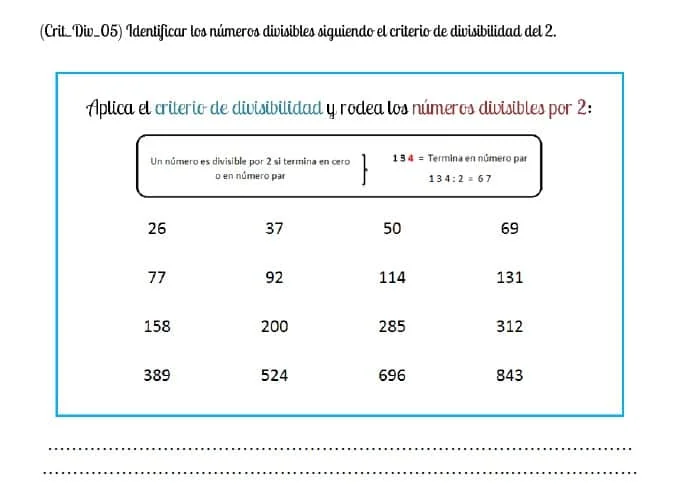#### Know and apply the divisibility rule of 3

A natural number is divisible by 3 if the result of the sum of all its digits is a multiple of 3, that is: 3, 6, 9, 12, 15, 18, 21, 24, 27...

Example: 3 1 5 = 3 + 1 + 5 = 9 and, therefore, it will be divisible by 3.

SPANISHENGLISH#### Know and apply the divisibility rule of 4

A natural number is divisible by 4 if it meets either of the two conditions:

a) Its last two digits are 00

Example: The natural number 700 is divisible by 4 because its last two digits (tens and ones) end in 0.

SPANISHENGLISHb) Its last two digits are a multiple of 4, for example 04, 08, 12, 16, 20, 24, 28, 32, 36, 40, 44, 48, 52, 56, 60, 64, 68, 72 , 76, 80, 84, 88, 92, 96, 100, 104, 108, 112, 116, 120...

Example: The number 7. 5 3 6 is divisible by 4 because its last two digits are 36, which is a multiple of 4 because 36/4 = 9, resulting in an exact division.

#### Know and apply the divisibility rule of 5

A natural number is divisible by 5 if the value of its last digit (ones place) is zero or five.

Example: 2 2 0 ends in zero and is therefore divisible by 5.

SPANISHENGLISH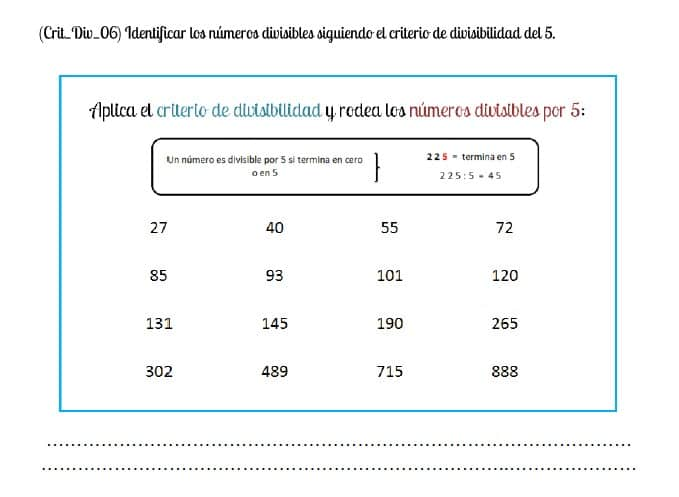#### Know and apply the divisibility rule of 6

A natural number is divisible by 6 when the divisibility criteria of both 2 and 3 are met simultaneously:

- A natural number is divisible by 2 if it ends in zero or even number, that is, 0, 2, 4, 6 or 8.

Example: 5 8 2 ends in two (even number) and is therefore divisible by 2.

SPANISHENGLISH- A natural number is divisible by 3 if the result of the sum of all its digits is a multiple of 3, that is: 3, 6, 9, 12, 15, 18, 21, 24, 27...

Example: 5 8 2 = 5 + 8 + 2 = 15 and, therefore, it will be divisible by 3.

SPANISHENGLISHTherefore, we can affirm that the number 582 is divisible by 6 when both the divisibility criteria of 2 and 3 are met simultaneously

#### Know and apply the divisibility rule of 7

A natural number is divisible by 7 when the difference between the number without the ones digit and twice the ones digit is zero or a multiple of 7, that is, 7, 14, 21, 28, 35, 42, 49, 56, 63, 70, 77, 84, 91, 98, 105, 112, 119, 126, 133, 140, 147...

Example: 5 4 6 = 5 4 - ( 2 x 6 ) = 5 4 - 1 2 = 42, which is a multiple of 7 because 42 : 7 = 6 (exact division)

Example: 3. 6 8 2 = 3 6 8 - ( 2 x 2 ) = 3 6 8 - 4 = 364, which is a multiple of 7 because 364 : 7 = 52 (exact division)

SPANISHENGLISH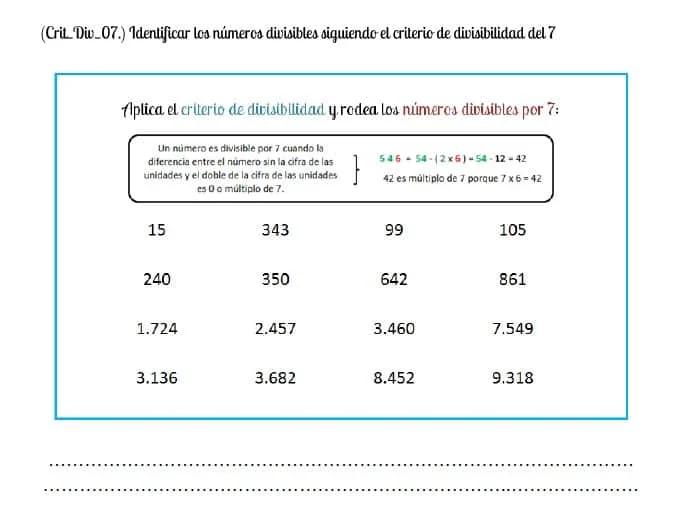#### Know and apply the divisibility rule of 8

A natural number is divisible by 8 if it meets either of the two conditions:

a) Its last three digits are 000

Example: The natural number 14.000 is divisible by 8 because its last three digits (hundreds, tens, and ones) end in 0.

SPANISHENGLISHb) Its last three digits are a multiple of 8, for example, 008, 016, 024, 032, 040, 048, 056, 064, 072, 080, 088, 096, 104, 112, 120, 128, 136...

Example: The number 6.112 is divisible by 8 because its last three digits are 112, which is a multiple of 8 because 112/8 = 14, resulting in an exact division.

#### Know and apply the divisibility rule of 9

A natural number is divisible by 9 if the result of the sum of all its figures is a multiple of 9, that is: 9, 18, 27, 36, 45, 54, 63, 72, 81...

Example: 3. 5 4 6 = 3 + 5 + 4 + 6 = 18 and, therefore, it will be divisible by 9 because 18/9 = 2 (exact division)

SPANISHENGLISH#### Know and apply the divisibility rule of 10

A natural number is divisible by 10 if its last digit (ones place) is zero.

Example: 5 7 0 ends in zero and is therefore divisible by 10.

SPANISHENGLISH#### Know and apply the divisibility rule of 11

A natural number is divisible by 11 when the sum of the odd position digits, minus the sum of the even position digits, is zero or a multiple of 11, that is, 11, 22, 33, 44, 55, 66, 77, 88, 99, 110, 121, 132, 143, 154, 165, 176, 187 ...

Example: 5 9 . 6 9 7 = ( 5 + 6 + 7 ) - ( 9 + 9 ) = 0

Example: 5 6. 7 1 6 = (5 + 7 + 6) - (6 + 1) = 18 - 7 = 11, which is a multiple of 11

SPANISHENGLISH#### Know and apply the divisibility rule of 12

A natural number is divisible by 12 when the divisibility criteria of both 3 and 4 are met in parallel:

- A natural number is divisible by 3 if the result of the sum of all its digits is a multiple of 3, that is: 3, 6, 9, 12, 15, 18, 21, 24, 27...

Example: 4 6 8 = 4 + 6 + 8 = 18 and, therefore, it will be divisible by 3.

SPANISHENGLISH- A natural number is divisible by 4 if its last two digits are 00 (for example, 1,800) or a two-digit number multiple of 4: 04, 08, 12 , 16, 20, 24, 28, 32, 36, 40, 44, 48, 52...

Example: 4 6 8, its last two digits are 68, which is a multiple of 4 because 68 / 4 = 17 and, therefore, the number 468 is divisible by 4.

SPANISHENGLISHTherefore, we can affirm that the number 468 is divisible by 12 when both the divisibility criteria of 3 and 4 are met simultaneously

#### Know and apply the divisibility rule of 13

A natural number is divisible by 13 when, when separating the digit from the ones place, multiplying it by 9 and subtracting it from the remaining digits (tens, hundreds, thousands, ten thousands...), the result is the same to 0 or a multiple of 13: 13, 26, 39, 52, 65, 78, 91, 104, 117, 130, 143, 156, 169, 182, 195...

Example: 5 4 6 = 5 4 - (6 x 9) = 5 4 - 5 4 = 0, therefore 546 will be divisible by 13

Example: 1. 7 1 6 = 1 7 1 - (6 x 9) = 1 7 1 - 5 4 = 117, which is a multiple of 13 because 117/13 = 9 (exact division) and therefore 1,716 will be divisible by 13

#### Know and apply the divisibility rule of 15

A natural number is divisible by 15 when the divisibility criteria of both 3 and 5 are met synchronously:

- A natural number is divisible by 3 if the result of the sum of all its digits is a multiple of 3, that is: 3, 6, 9, 12, 15, 18, 21, 24, 27...

Example: 3 1 5 = 3 + 1 + 5 = 9 and, therefore, it will be divisible by 3.

SPANISHENGLISH- A natural number is divisible by 5 if the value of its last digit (ones place) is zero or five.

Example: 3 1 5 ends in five and is therefore divisible by 5.

SPANISHENGLISHTherefore, we can affirm that the number 315 is divisible by 15 when both the divisibility criteria of 3 and 5 are met simultaneously

#### Find the prime and composite numbers of any natural number

A number is prime when it only has two factors (divisors), itself and unity.

Example: The number 7 is a prime number because it can only be divided by 1 (the unit) and by 7 (by itself)

SPANISHENGLISHOn the other hand, a number is composite when it has more than two factors:

Example: The number 10 is a composite number because it can be divided by 1 (the unit), 2, 5 and 10 (by itself)

And remember! The number 1 is neither prime nor compound because it only has one divisor, itself.

The Greek mathematician Eratosthenes of Cyrene (276 - 194 BC) drew up a table with the prime and composite numbers from 1 to 100 which he called the "sieve of Eratosthenes".

Would you be able to replicate it? We propose some activities for you to achieve it!#### Calculate the multiples of a natural number

The multiples of any natural number are obtained by multiplying said number by 0, 1, 2, 3, 4... that is, following its multiplication table in an orderly manner and with a tendency towards infinity.

Do not forget! The number 0 is a multiple of all numbers.

SPANISHENGLISHWe provide you the lesson so that you can learn what multiples are and how to find them from any natural number: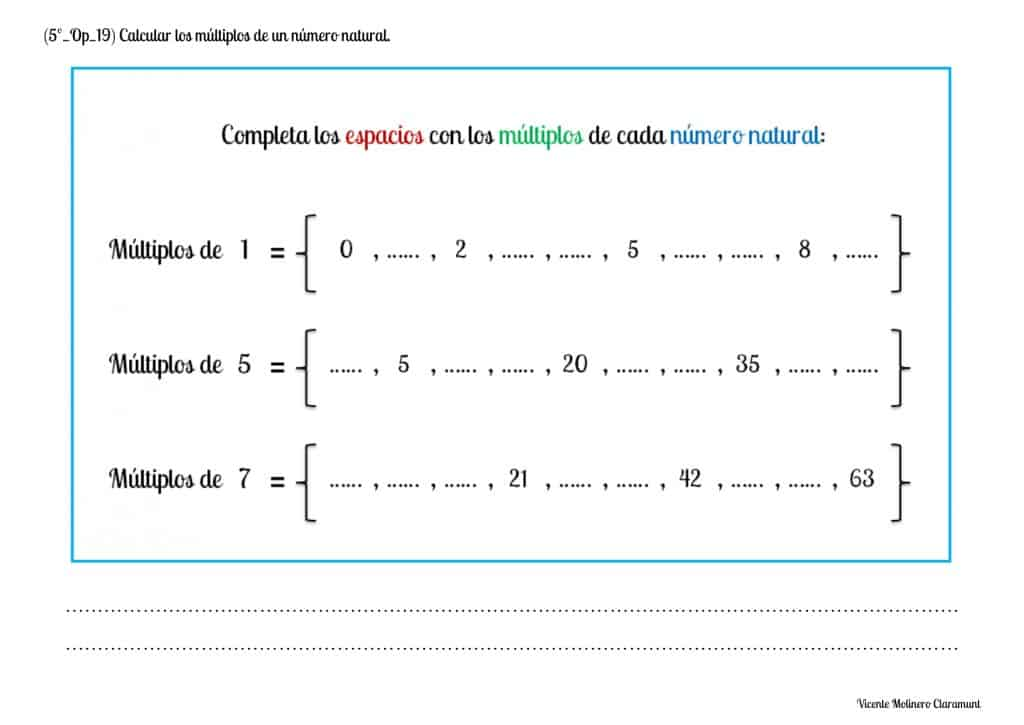#### Calculate the factors (divisors) of a natural number

A number is factor of another if, when dividing by each other, the division is exact with remainder zero.

And remember! The number 1 is a factor of all numbers.

SPANISHENGLISHWe provide you the explanation so that you can learn what factors (divisors) are and how to find them from any natural number.

If we want to know what are the divisors of any natural number we would have to divide all the numbers from 1 to the number we want to reach (in this exemple, 8) and we would consider factors those in which the division was exact: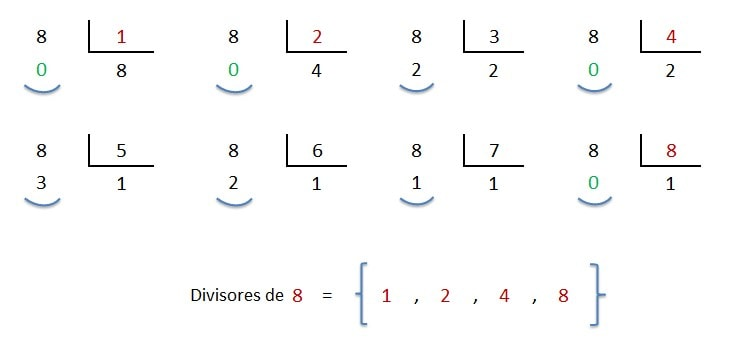As you may have seen, finding the factors of a number can be an expensive task if we use larger numbers and, for this reason, we are going to teach you the most used method to calculate the divisors of any natural number quickly and easily:

DECOMPOSITION BY FACTORIZATION OF PRIME NUMBERS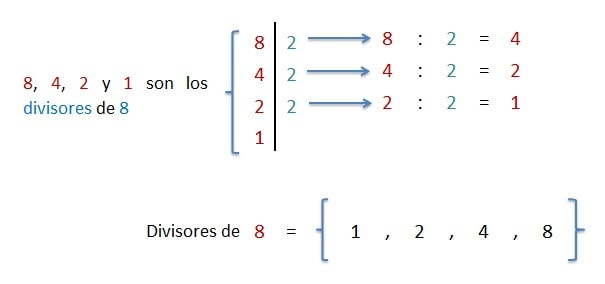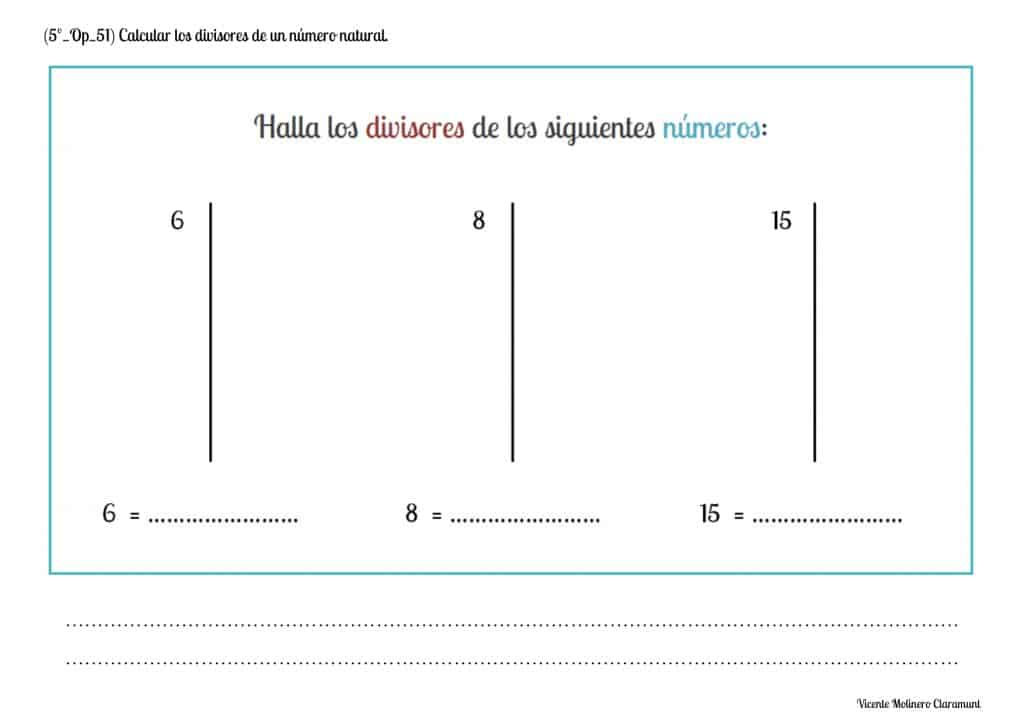#### Find the Least Common Multiple (LCM) of two given natural numbers

We present you a series of printable activities, a tutorial and an educational game so that you understand what the Least Common Multiple (LCM) is and also easily learn to calculate it between two natural numbers.

SPANISHENGLISHNext, we show you the two existing procedures for obtaining the Least Common Multiple of two natural numbers: the "LCM by Listing Method" and the "LCM using Prime Factorization":

LCM OF TWO NUMBERS BY LISTING METHOD

We will find the multiples of the two natural numbers by following their multiplication tables in order. The Least Common Multiple will be the smallest of the common multiples: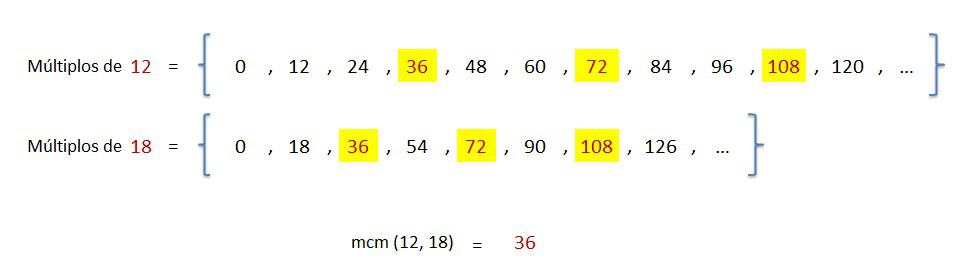LCM OF TWO NUMBERS USING PRIME FACTORIZATION

Starting from two or more natural numbers and through their decomposition expressed as the product of prime factors (that is, 2, 3, 5, 7, 11, 13...), the Least Common Multiple (LCM) will be found by multiplying all common and uncommon factors raised to their greatest exponent: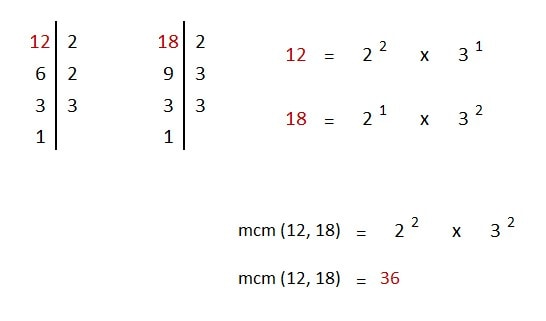#### Find the Least Common Multiple (LCM) of three given natural numbers

We present some worksheets in PDF format along with their explanation so that you understand step by step how to find the Least Common Multiple (LCM) of three natural numbers.

SPANISHENGLISHLCM OF THREE NUMBERS BY LISTING METHOD

We will find the multiples of the three natural numbers by following their multiplication tables in order. The Least Common Multiple will be the smallest of the common multiples :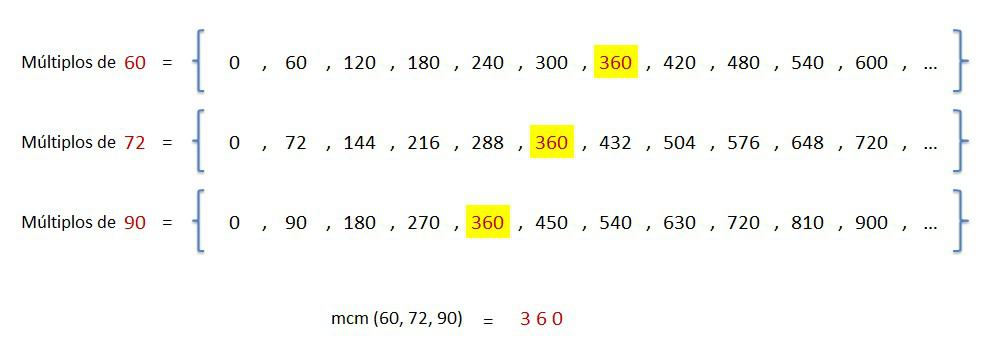LCM OF THREE NUMBERS USING PRIME FACTORIZATION

Starting from two or more natural numbers and through their decomposition expressed as the product of prime factors (that is, 2, 3, 5, 7, 11, 13...), the Least Common Multiple (LCM) will be found by multiplying all common and uncommon factors raised to their greatest exponent: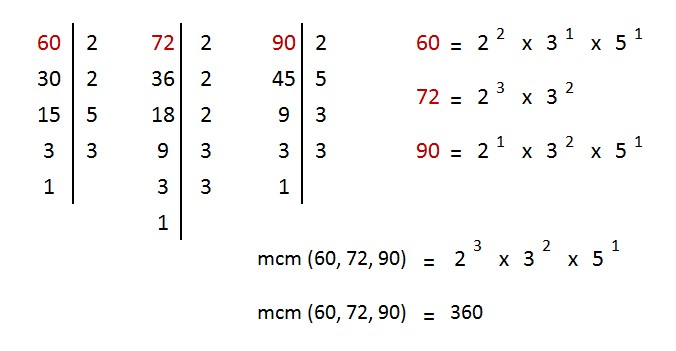#### Find the Greatest Common Factor (GCF) of two given natural numbers

Watch the explanatory video and you will learn how to find the Greatest Common Factor (GCF) of two natural numbers. Then practice with the online activity and with our downloadable worksheets.

SPANISHENGLISHGCF OF TWO NUMBERS USING PRIME FACTORIZATION

Starting from two or more natural numbers and through their decomposition expressed as the product of prime factors (that is, 2, 3, 5, 7, 11, 13...), the Greatest Common Factor (GCF) will be found by multiplying all the common factors raised to their lowest exponent: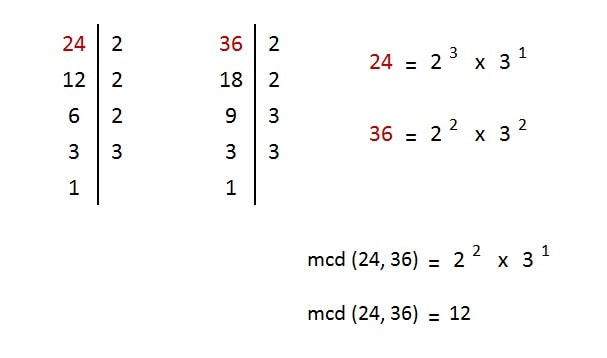The Greek mathematician and geometrist Euclid of Alexandria (325 - 265 BC), also known as the "father of geometry", developed an algorithm to find the Greatest Common Factor of two natural numbers:

- If when dividing the greater number by the smaller number the division is exact, the GCF will be the value of the divisor.

- If when dividing the greater number by the smaller number the division is inexact, the GCF will be the value of the remainder.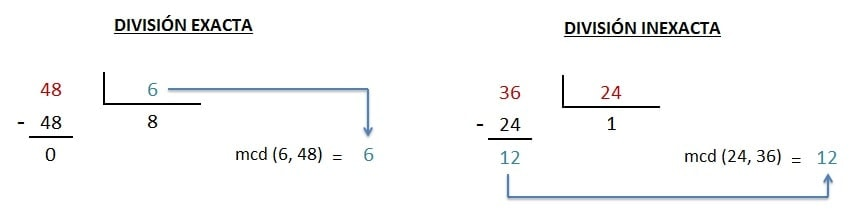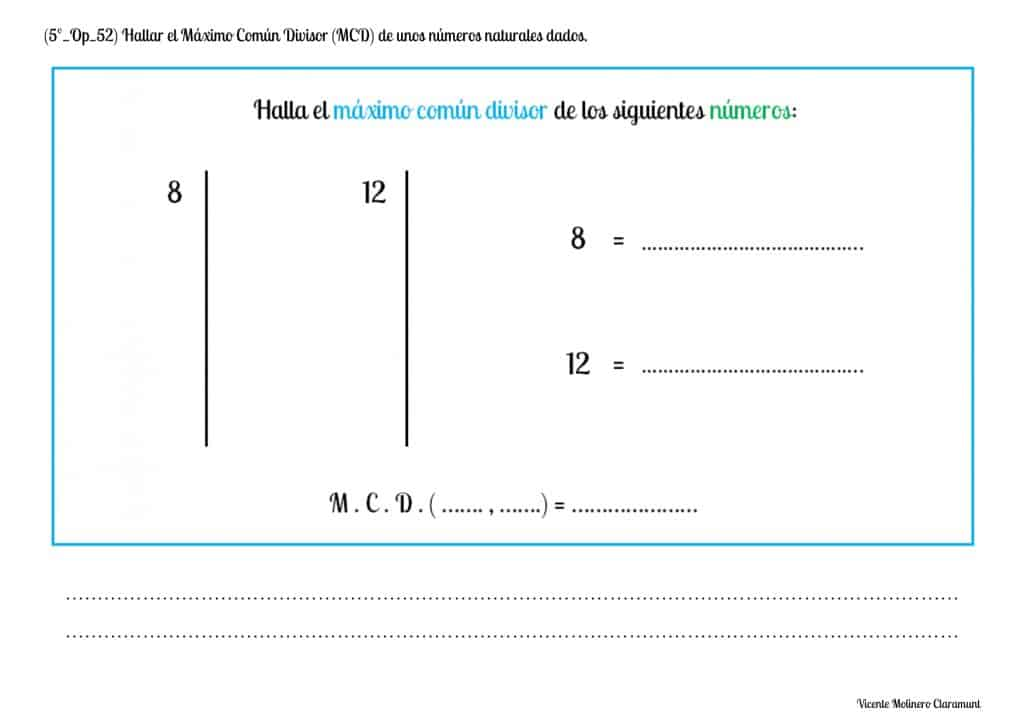#### Find the Greatest Common Factor (GCF) of three given natural numbers

We present some exercises in PDF format along with their explanation so that you understand step by step how to find the Greatest Common Factor (GCF) of three natural numbers.

SPANISHENGLISHGCF OF THREE NUMBERS USING PRIME FACTORIZATION

Starting from three or more natural numbers and through their decomposition expressed as the product of prime factors (that is, 2, 3, 5, 7, 11, 13...), the Greatest Common Factor (GCF) will be found by multiplying all the common factors raised to their lowest exponent: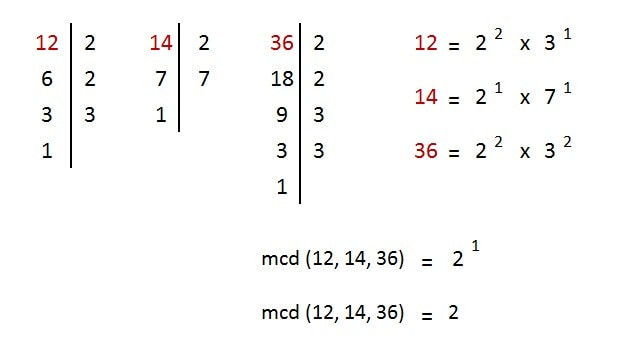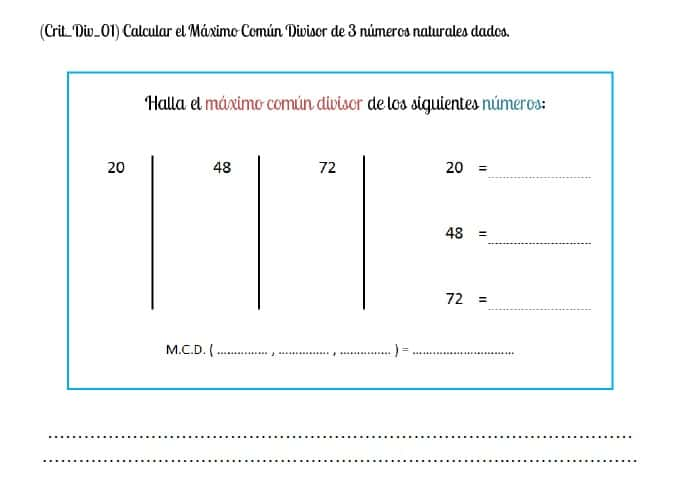If you are not willing to learn, no one can help you; but if you have the firm conviction to learn... no one can stop you

Helen Keller